# The easiest word problems

#### Number of problems found: 7295

• School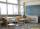27 students of 6.D class are going on a trip. They pay 9 € each. The teacher got 153 €. How many children have not paid?
• Cycling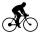Cyclist goes on a trip. First, drove 4 hours average speed 44 kilometers per hour, then slowed down and another 4 hours driving a speed 36 kilometers per hour. How many total kilometers cycling?
• Winnie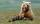Winnie Hugo had the right paw 10 punches and on 15 left more. How many punches has on left paw?
• Cow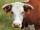104 cow consumes 6968 kg hay per day. How many kg of hay consume one cow in one day?
• Vinegar 2How many percentages of vinegar solution will we get if we mix 3.5 liters of 5.8% and 5 liters of 7.6% vinegar?
• Derivation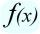Exists a function whose derivation is the same function?
• Triangle P2Can a triangle have two right angles?
• SequenceWrite the first 7 members of an arithmetic sequence: a1=-3, d=6.
• Painter Pavel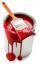Painter Pavel painted the fence for 16 hours and painter Petr the same fence painted for 13 h. How long should take painted the fence together?
• MilimetersHow many millimeters are 1/4 meters?
• DigitsWrite the smallest and largest 2-digit natural number.
• Trapezoid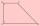Are diagonals in a rectangular trapezoid perpendicular and bisect the angles?
• Cakes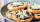On the table was 56 cakes. When Peter went home, leaving them eight times less. How many cakes left?
• DiscountLadies sweater was twice discounted. First by 8%, then by 8% of the new price. Its final price was 66 €. Determine the original price of the sweater.
• Hours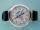How many hours is 9 days?
• Computer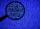A print line on a computer contains 64 characters (letters, spacers, or other chars). Find how many characters there are in 7 lines.
• Percent calculationCalculate 8% if 44% is 32.Between cities A and B is route 13 km long of stúpanie average 7‰. Calculate the height difference of cities A and B.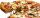Master says that he can splits pizza to 16 parts by five equals straight cuts. Is it possible?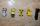Peter has 29 toy cars, Zdenko has 8 toy cars less. How many toy cars has Zdenko?